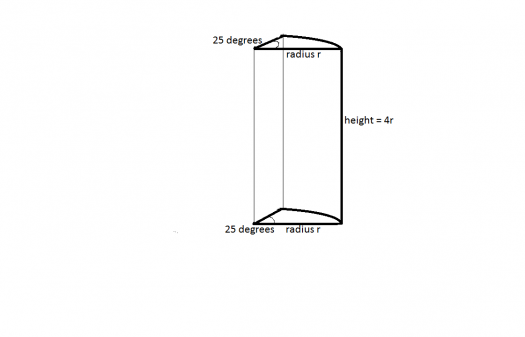# prism ..solid mensuration

2 posts / 0 new
Jhomar A Españolprism ..solid mensuration

find the height of a prism with a base in the form of a sector in which the central angle is 25. its volume is found to be 350in.3 . and the height is four times the radius of the base. - See more at: /forum/strength-materials/need-help#comment-8050

fitzmerl duronTo get the height of this weird cylinder, recall the formula:

$$V=BH$$

where $V$ is the volume of the figure, $B$ is the area of the base, and $H$ is the height of the figure.With that in mind, we plug this given to the formula above:

$$V=BH$$ $$350 \space in^3 = \left(\frac{1}{2}r^2\theta\right)(4r)$$
$$350 \space in^3 = \left(\frac{1}{2}(r^2)\left(25^o\left(\frac{\pi}{180^o}\right)\right)\right)(4r)$$ $$350 \space in^3 = \left(\frac{1}{2}(r^2)\left(\frac{5\pi}{36}\right)\right)(4r)$$ $$350 \space in^3 = \frac{5\pi}{18}r^3$$ $$\frac{1260}{\pi} = r^3$$ $$r = 7.3 \space inches$$

Note that the area of a sector of circle is $A=\frac{1}{2}r^2\theta$, where $r$ is the radius of the sector and $\theta$ is the central angle of the sector.

Since the problem asks for the height of this weird cylinder, which is four times longer than the radius of the sector of circle, so:
$$H = 4r$$ $$H = 4(7.3 \space in)$$ $$H = 29.2 \space in$$

Therefore, the height of this weird cylinder is $\color{green}{H=29.2 \space in}$.

Alternate solutions are encouraged...

Subscribe to MATHalino on